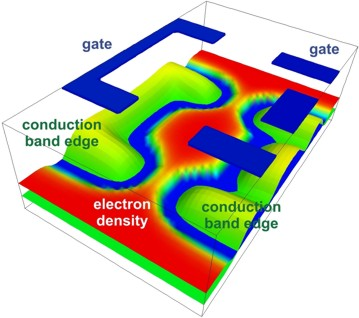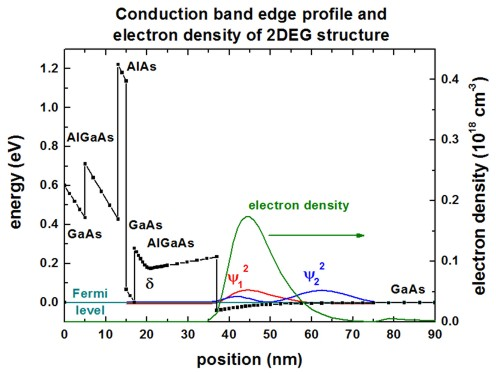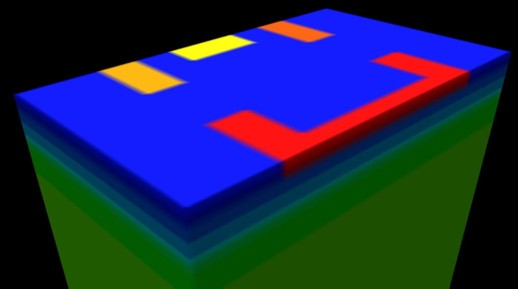nextnano.com  GUI: nextnanomat  Tool: nextnano++  Tool: nextnano³  Tool: nextnano.NEGF  Download | Search | Copyright | News | Publications  * password protected nextnano³ software3D SET

# nextnano3 - Tutorial

## Single-electron transistor - laterally defined quantum dot

Author: Stefan Birner

If you want to obtain the input files that are used within this tutorial, please contact stefan.birner@nextnano.de.
```-> SET_Scholze_IEEE2000_1D.in            / *_nnp.in - ```input file for the nextnano3 and nextnano++ software
```-> SET_Scholze_IEEE2000_3D.in            / *_nnp.in - ```input file for the nextnano3 and nextnano++ software``` -> SET_Scholze_IEEE2000_3D_top_gates.in  / *_nnp.in - ```input file for the nextnano3 and nextnano++ software``` ```

## Single-electron transistor - laterally defined quantum dot

In this tutorial, we simulate an AlGaAs/GaAs heterostructure grown along the z direction.
This structure leads to a two-dimensional electron gas (2DEG).
By appying a gate voltage on top of the structure in the (x,y) plane, one is able to deplete the 2DEG and a laterally defined QD is formed.
By adjusting the gate voltage, one is able to tune the number of electrons that are inside the QD.This figure shows the conduction band edge Ec(x,y) and the electron density n(x,y) for the 2DEG plane, i.e. at z = 8 nm below the GaAs/AlGaAs heterojuntion.
The geometry of the top gates is indicated by the blue regions.

We divide the tutorial into two parts:

• In part 1, we simulate the heterostructure along the z direction and neglect the gates.
(1D simulation: self-consistent Schrödinger-Poisson equation)

`-> SET_Scholze_IEEE2000_1D.in`
• In part 2, we solve the 3D Poisson equation for the same structure as in part 1
(3D simulation: self-consistent Schrödinger-Poisson equation)

```-> SET_Scholze_IEEE2000_3D.in ```
• In part 3, we solve the 3D Poisson equation to study the effect of the gates.
(3D simulation: only Poisson equation using a classical density

```-> SET_Scholze_IEEE2000_3D_top_gates_cl.in ```
• In part 4, we solve the 3D Schrödinger-Poisson equation.
(Part 4 is not documented yet.)

`-> SET_Scholze_IEEE2000_3D_top_gates_qm.in`

The tutorial is based on the following paper:

Single-Electron Device Simulation
A. Scholze, A. Schenk, W. Fichtner
IEEE Transactions on Electron Devices 47, 1811 (2000)

## Part 1: 1D simulation (self-consistent Schrödinger-Poisson)

The following figure shows the calculated conduction band edge and the electron density of the heterostructure.
The results are similar to Fig. 4 of the above mentioned paper.At the left boundary, a Schottky barrier of 0.6 V has been assumed.
At z = 20 nm, a delta doping layer is present.
The Fermi level is assumed to be constant at EF = 0 eV.
The ground state wave function (psi1) is ~8 meV below the Fermi level and dominates the electron density.
The first excited state (psi2) is ~3 meV above the Fermi level, the second excited state (not shown) is 19 meV above the Fermi level.

## Part 2: 3D simulation of Part 1 (self-consistent Schrödinger-Poisson equation)

(to do: add comments and figures...)

## Part 3: 3D simulation with top gates (Poisson equation only)

The following figure shows the 3D structure that we are going to simulate.The following figure shows two 2D slices through the lateral (x,y) plane at a distance of 8 nm below the AlGaAs/GaAs interface.
- In the middle, the electron density is shown.
The electron density has been calculated classically.
- At the bottom, the conduction band edge is shown.
The results are similar to Fig. 5 of the above mentioned paper.
At the top, the four gates are shown.## Part 4: 3D simulation with top gates (self-consistent Schrödinger-Poisson equation)

(to do: add comments and figures...)

• Please help us to improve our tutorial. Send comments to``` support [at] nextnano.com```.